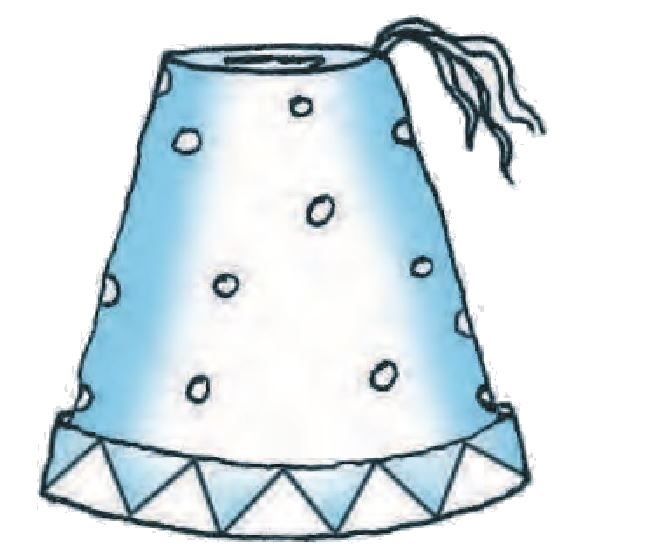# Ex.13.4 Q3 Surface Areas and Volumes Solution - NCERT Maths Class 10

Go back to  'Ex.13.4'

## Question

A fez, the cap used by the Turks, is shaped like the frustum of a cone (see Fig. 13.24). If its radius on the open side is $$10\,\rm{cm}$$, radius at the upper base is $$4\,\rm{cm}$$ and its slant height is $$15\,\rm{cm, }$$ find the area of material used for making it.

Video Solution
Surface Areas And Volumes
Ex 13.4 | Question 3

## Text Solution

What is Known?

A fez is shaped like the frustum of a cone with radius of open side $$10\,\rm{cm},$$

radius at the upper base $$4\,\rm{cm}$$ and Slant Height $$15 \,\rm{cm}$$

What is Unknown?

Area of the material used for making Fez

Reasoning:Since the fez is in the shape of frustum of a cone and is open at the bottom.

Therefore,

Area of material used for making fez $$=$$ Curved Surface Area of the frustum $$+$$ Area of the upper circular end

We will find the Area of material by using formulae;

CSA of frustum of a cone $$= \pi \left( {{r_1} + {r_2}} \right)l$$

where $$r1, r2$$ and $$l$$ are the radii and slant height of the frustum of the cone respectively.

Area of the circle $$= \pi {r^2}$$

where $$r$$ is the radius of the circle.

Steps:

Slant height, $$l =15\,\rm{cm}$$

Radius of open side $$r_1=10\,\rm{cm}$$

Radius of upper base $${r_2} =4\,\rm{cm}$$

Area of material used for making fez $$=$$ Curved Surface area of the frustum $$+$$ area of the upper circular end

\begin{align}&= \pi \left( {{r_1} + {r_2}} \right)l + \pi {r^2}\\&= \pi \left[ {\left( {{r_1} + {r_2}} \right)l + r_2^2} \right]\end{align}

\begin{align}&=\!\frac{{22}}{7}\left[\!{\left( {10cm\!+\!4cm} \right)\!15cm\!+\!\!{{\left( {4cm} \right)}\!^2}}\!\right]\\&= \frac{{22}}{7}\left[ {14cm \times 15cm + 16c{m^2}} \right]\\&= \frac{{22}}{7}\left[ {210c{m^2} + 16c{m^2}} \right]\\&= \frac{{22}}{7} \times 226c{m^2}\\&= \frac{{4972}}{7}c{m^2}\\&= 710\frac{2}{7}c{m^2}\end{align}

\begin{align} 710\frac{2}{7}c{m^2}\end{align} of the material used for making Fez.

Learn from the best math teachers and top your exams

• Live one on one classroom and doubt clearing
• Practice worksheets in and after class for conceptual clarity
• Personalized curriculum to keep up with school